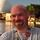## General Question# Brain Teaser (light) - How well do you understand weighted averages?

Asked by LostInParadise (28461) June 12th, 2009

Weighted averages are used frequently in engineering applications involving filtering and tracking, such as RADAR and neural network calculations, as well as determining school grades and estimating batting averages.

You should already have some intuitional understanding of weighted averages. For example, if a person taking a class has a score of 80 for 90% of the grade and a score of 95 for 10% of the grade you should know without calculation that the final grade will be between 80 and 95 and because the 90% factor is greater, the score will be closer to 80 than 95, so the final grade is between 80 and 87.5. I hope that most of you know that the calculation of the grade is .90*80 + .10*95 = 81.5.

Now consider the following problem. A person’s grade in a class is based on the scores on a quiz, a midterm and a final, with scores respectively of 80, 90 and 94. The final counts more than the midterm and the midterm counts more than the quiz. What can you say about the final grade? We know that it will be between 80 and 94 but a stronger statement can be made.

Observing members: 0Composing members: 0## 2 AnswersThe final grade is greater than 88 (which would be the grade if they were all waited equally. Since the higher grades are both worth more than the lower grade, the final grade must be greater than that).

The final grade is less than or equal to 93.96 (assuming the percentages are whole percentage points, not decimals). This figure assumes the quiz is worth 0%, the midterm worth 1%, and the final worth 99%.

So I’m sure we can say the final grade is greater than 88 and less than 93.96. I’m willing to bet we can say more than that, but this is as far as I get right now….

MrItty (17381)“Great Answer” (0) Flag as…@MrItty , I had not thought about working with an upper bound, but I like your thinking on that. If you assume that the quiz is worth something and that the weightings are in increments of 5%, then you could conjecture weightings of 5%, 10% and 85%, which would put the score between 88 and 92.9.

LostInParadise (28461)“Great Answer” (0) Flag as…## Answer this questionor

#### Join

to answer.

This question is in the General Section. Responses must be helpful and on-topic.

Your answer will be saved while you login or join.
Have a question? Ask Fluther!
What do you know more about?
or
Knowledge Networking @ Fluther